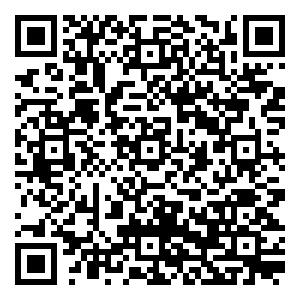2.793

2018影响因子

(CJCR)

• 中文核心
• EI
• 中国科技核心
• Scopus
• CSCD
• 英国科学文摘

## 留言板引用本文: 路遥. 非仿射高超声速飞行器输出反馈控制方法研究. 自动化学报, 2021, x(x): 1−13Lu Yao. A study on output feedback control for non-affine hypersonic vehicles. Acta Automatica Sinica, 2021, x(x): 1−13
 Citation: Lu Yao. A study on output feedback control for non-affine hypersonic vehicles. Acta Automatica Sinica, 2021, x(x): 1−13## A Study on Output Feedback Control for Non-affine Hypersonic Vehicles

Funds: Supported by National Natural Science Foundation of P. R. China (No.61803357)
###### Author Bio: LU Yao　senior engineer at the Beijing aerospace automatic control institute. His research interest is nonlinear control theory and its application in flight control
• 摘要: 针对一类考虑模型非仿射特性和执行机构饱和特性的高超声速飞行器轨迹跟踪控制问题, 提出一种基于backstepping的输出反馈非线性控制方法. 考虑执行机构故障激发的未知非线性动态, 建立了非仿射形式飞行器模型. 为解决实际工程应用中存在的气流角测量值难以使用的问题, 利用高度和速度测量值以及高阶微分器设计了航迹倾角在线估计方法. 基于跟踪微分器设计了模型干扰项的估计方法, 并解决了backstepping方法应用中存在的“微分项爆炸”问题. 引入辅助系统降低控制量饱和带来的不利影响. 基于Lyapunov理论证明了闭环系统的稳定性. 最后, 通过对比仿真实验验证了所提方法的有效性.
• 图  1  控制器结构图

Fig.  1  Structure diagram of the controller

图  2  速度跟踪曲线

Fig.  2  Tracking curves of velocity

图  13  本文方法抗饱和补偿信号曲线

Fig.  13  Curves of anti-windup compensated signals under proposed method

图  3  航迹倾角跟踪曲线

Fig.  3  Tracking curves of flight path angle

图  4  速度跟踪误差曲线

Fig.  4  Curves of velocity tracking errors

图  5  控制量燃料空气混合比曲线

Fig.  5  Curves of the control variable fuel-to-air ratio

图  7  俯仰角曲线

Fig.  7  Curves of pitch angle

图  6  航迹倾角跟踪误差曲线

Fig.  6  Curves of flight path angle tracking errors

图  8  俯仰角速度曲线

Fig.  8  Curves of pitch rate

图  9  航迹倾角估计误差曲线

Fig.  9  Curves of the estimated error of flight path angle

图  10  控制量升降舵摆角曲线

Fig.  10  Curves of the control variable deflection of elevator

图  11  本文方法航迹倾角子系统控制指令曲线

Fig.  11  Curves of flight path angle subsystem control commands under proposed method

图  12  本文方法总干扰项估计曲线

Fig.  12  Estimated curves of the lumped disturbances under proposed method

表  1  控制量的容许范围

Table  1  Admissible ranges for control inputs

 飞行状态/控制量 容许范围 $\phi$ [0.1,1.2] ${\delta _{\rm{e}}}$ [−15°,15°] ${\delta _{\rm{c}}}$ [−27°,27°]

表  2  控制器参数设置

Table  2  Parameter settings of controllers

 控制方法 控制器参数 本文方法 \begin{aligned} {k_V} = 5,{k_\gamma } = 1.5,{k_\theta } = {k_Q} = 5,{k_1} = 10,{k_2} = 10, \\ {R_H} = 100,{a_{H1} } = {a_{H2} } = 6,{a_{H3} } = 4,{R_V} = 100,\;\; \\ {R_\theta } = {R_{Qc} } = 10,{R_Q} = 50,{a_{V1} } = {a_{V2} } = 5,\;\;\;\; \qquad\\ {a_{\theta 1} } = {a_{Qc1} } = {a_{Q1} } = {a_{\theta 2} } = {a_{Qc2} } = {a_{Q2} } = 5.\qquad \end{aligned} 对比方法 \begin{aligned} { {k'}_1} = 5,{k_1} = 1.5,{k_2} = {k_3} = 5,\varepsilon = \varepsilon ' = 0.25, \;\;\;\;\;\\ \iota = \iota ' = 0.5,{M_0} = 15,{ {M'}_0} = 1.2, \qquad\qquad\qquad\;\;\;\;\\ {\mu _{i1} } = {\mu _{i2} } = 1,i = 2,3,{\theta _{j1} } = {\theta _{j2} } = 1,j = 1,2,3, \\ \theta _1^{'} = \theta _2^{'} = 10,\Delta {t_1} = \Delta {t_2} = \Delta {t_3} = 0.2.\qquad\qquad\;\; \end{aligned}
•点击查看大图
##### 计量
• 文章访问数:  137
• HTML全文浏览量:  178
• 被引次数: 0
##### 出版历程
• 收稿日期:  2021-02-07
• 录用日期:  2021-03-19
• 网络出版日期:  2021-05-20

### 目录/下载:  全尺寸图片 幻灯片
• 分享
• 用微信扫码二维码

分享至好友和朋友圈